# 【Python数据科学手册】专题：特征工程

+关注继续查看

### 1、分类数据

data = [
{'price': 850000, 'rooms': 4, 'neighborhood': 'Queen Anne'},
{'price': 700000, 'rooms': 3, 'neighborhood': 'Fremont'},
{'price': 650000, 'rooms': 3, 'neighborhood': 'Wallingford'},
{'price': 600000, 'rooms': 2, 'neighborhood': 'Fremont'}
]

{'Queen Anne': 1, 'Fremont': 2, 'Wallingford': 3};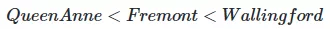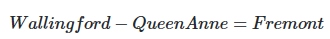from sklearn.feature_extraction import DictVectorizer
vec = DictVectorizer(sparse=False, dtype=int)
vec.fit_transform(data)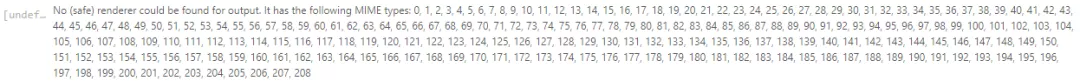neighborhood 字段转换成三列来表示三个地点标签，每一行中用1 所在的列对应一个地点。当这些分类特征编码之后，你就可以和之前一样拟合Scikit-Learn 模型了：

vec.get_feature_names()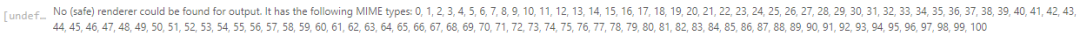vec = DictVectorizer(sparse=True, dtype=int)
vec.fit_transform(data)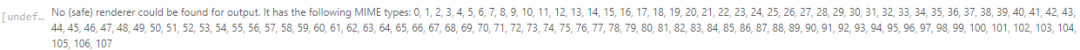### 2、文本特征

sample = ['problem of evil',
'evil queen',
'horizon problem']

from sklearn.feature_extraction.text import CountVectorizer

vec = CountVectorizer()
X = vec.fit_transform(sample)
X

import pandas as pd
pd.DataFrame(X.toarray(), columns=vec.get_feature_names())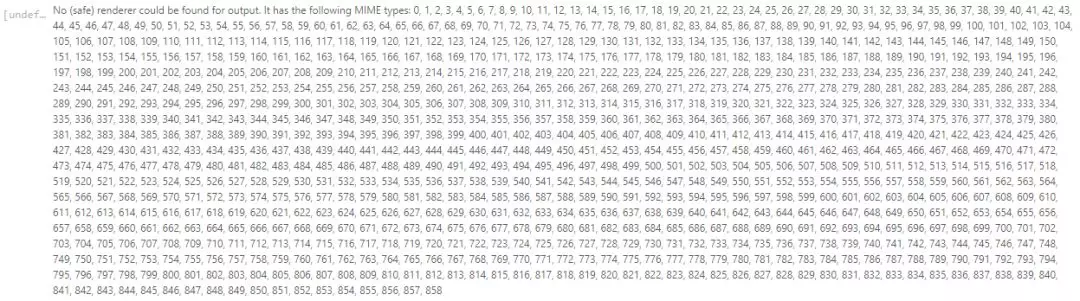from sklearn.feature_extraction.text import TfidfVectorizer
vec = TfidfVectorizer()
X = vec.fit_transform(sample)
pd.DataFrame(X.toarray(), columns=vec.get_feature_names())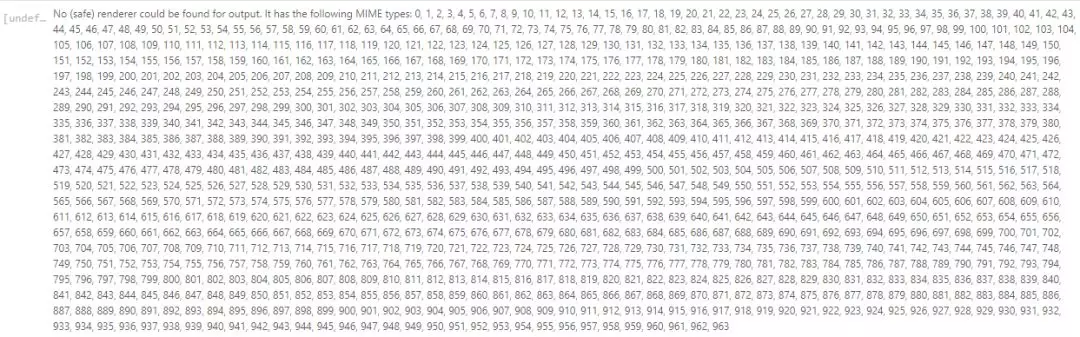### 4、衍生特征

%matplotlib inline
import numpy as np
import matplotlib.pyplot as plt

x = np.array([1, 2, 3, 4, 5])
y = np.array([4, 2, 1, 3, 7])
plt.scatter(x, y);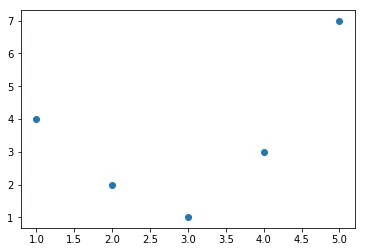from sklearn.linear_model import LinearRegression
X = x[:, np.newaxis]
model = LinearRegression().fit(X, y)
yfit = model.predict(X)
plt.scatter(x, y)
plt.plot(x, yfit);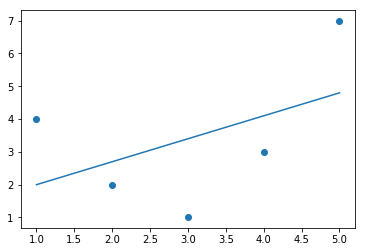from sklearn.preprocessing import PolynomialFeatures
poly = PolynomialFeatures(degree=3, include_bias=False)
X2 = poly.fit_transform(X)
print(X2)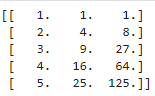model = LinearRegression().fit(X2, y)
yfit = model.predict(X2)
plt.scatter(x, y)
plt.plot(x, yfit);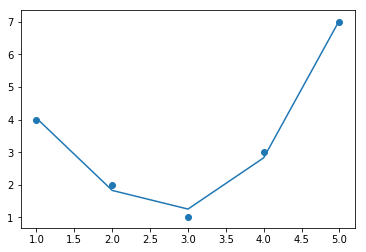### 5、缺失值填充

from numpy import nan
X = np.array([[ nan, 0,   3  ],
[ 3,   7,   9  ],
[ 3,   5,   2  ],
[ 4,   nan, 6  ],
[ 8,   8,   1  ]])
y = np.array([14, 16, -1,  8, -5])


from sklearn.preprocessing import Imputer
imp = Imputer(strategy='mean')
X2 = imp.fit_transform(X)
X2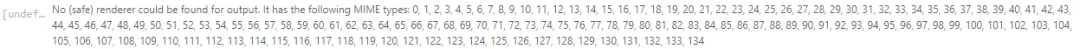model = LinearRegression().fit(X2, y)
model.predict(X2)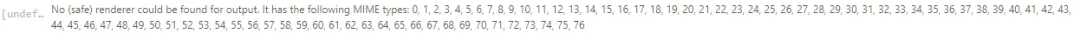### 6、特征管道

Scikit-Learn 提供了一个管道对象，如下所示：

from sklearn.pipeline import make_pipeline

model = make_pipeline(Imputer(strategy='mean'),
PolynomialFeatures(degree=2),
LinearRegression())

model.fit(X, y)  # X with missing values, from above
print(y)
print(model.predict(X))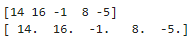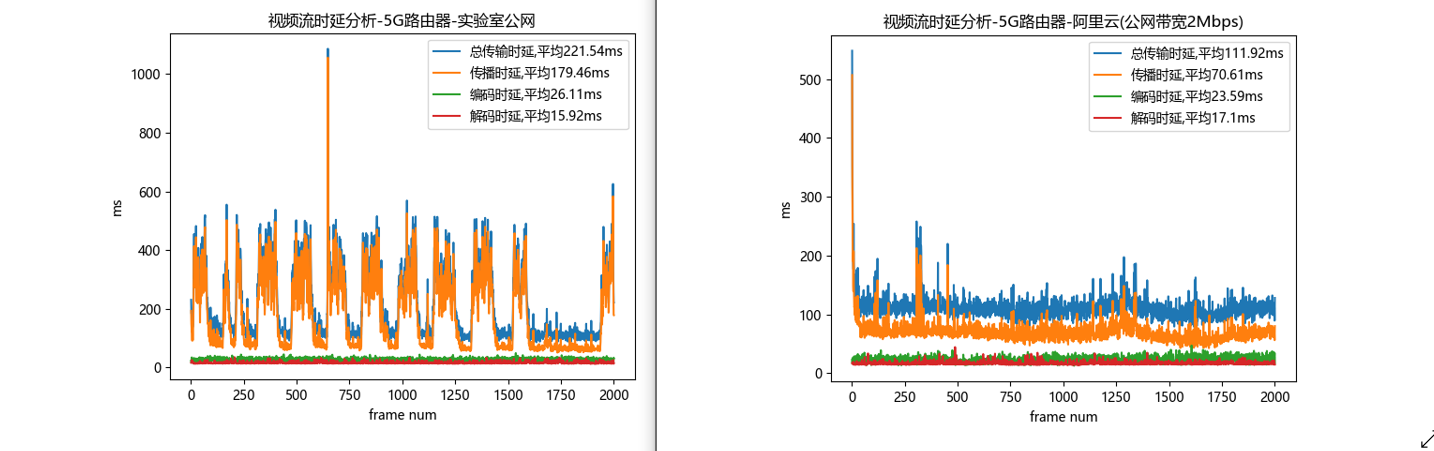19 03 0Python面试题大全（二）：python高级语法（下）
Python面试题大全（二）：python高级语法（下）
4 0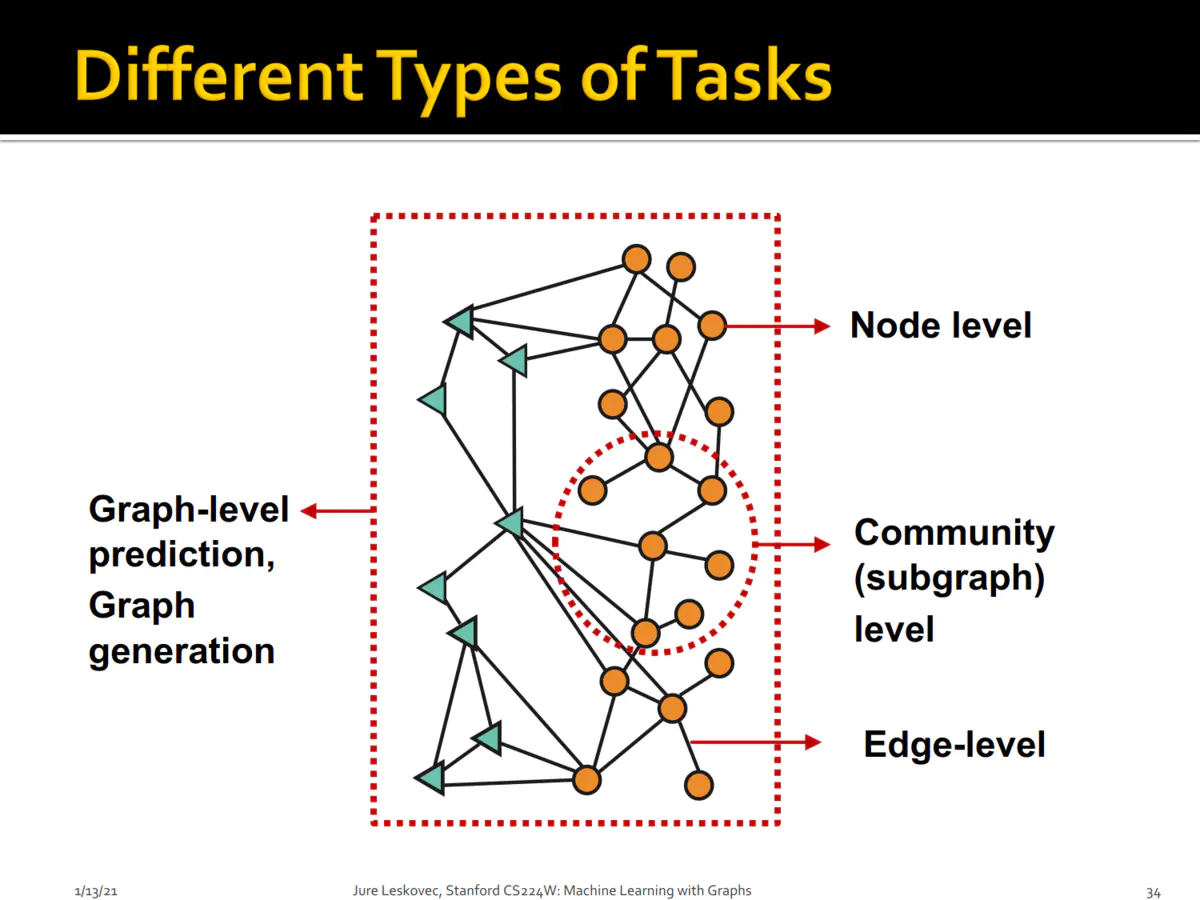4 0100道Python练习题，看看你能会几题！？（上）
100道Python练习题，看看你能会几题！？（上）
3 0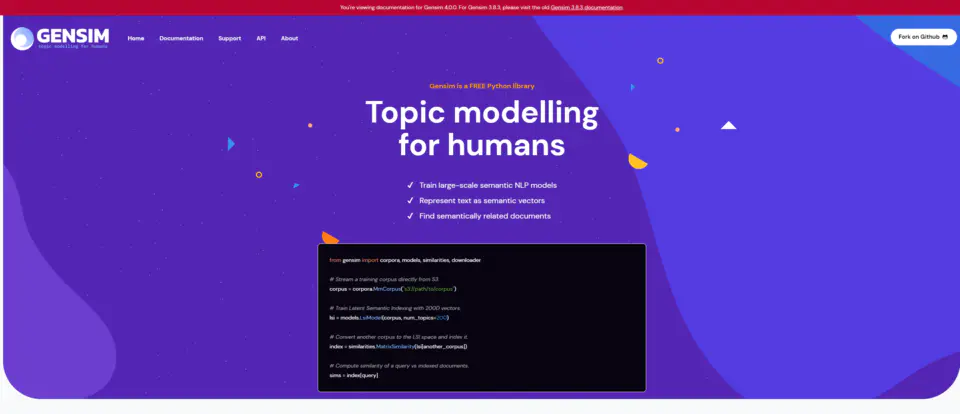5 0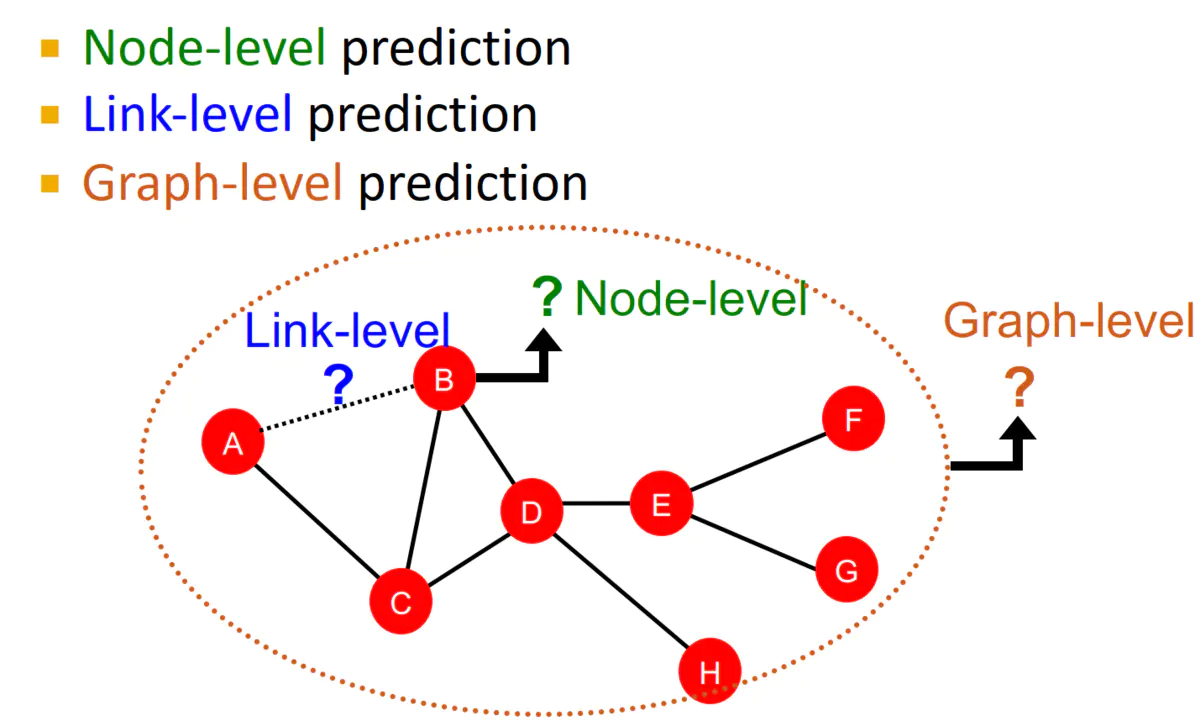2 011 0
770

584

+ 订阅

OceanBase 入门到实战教程